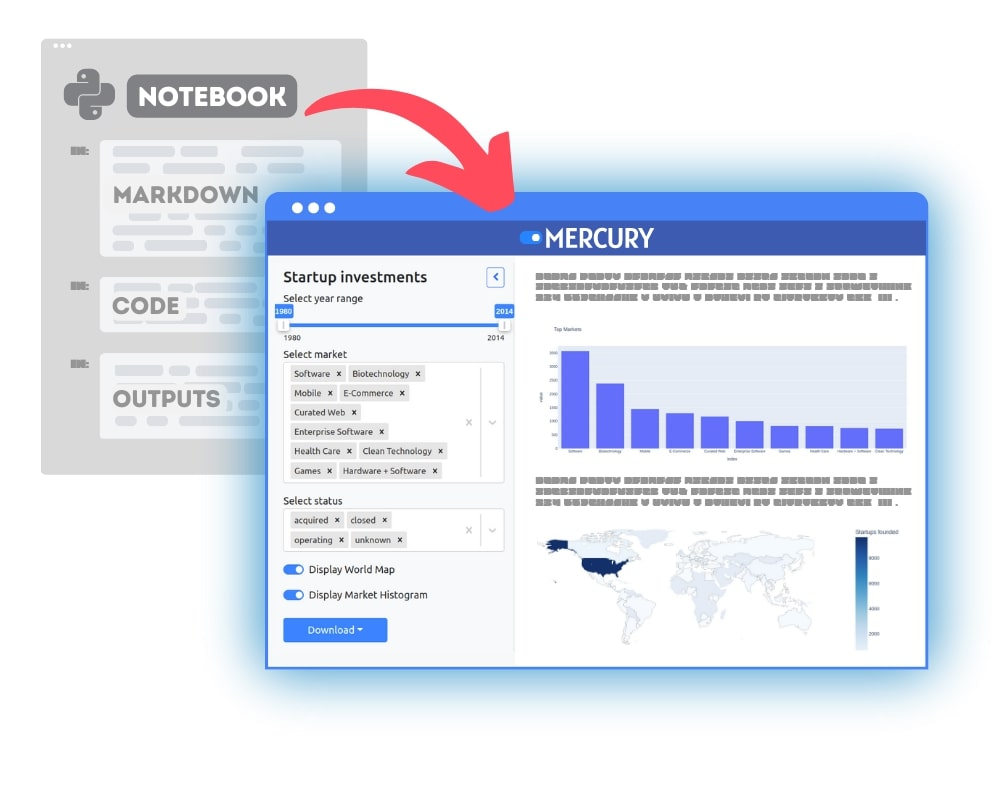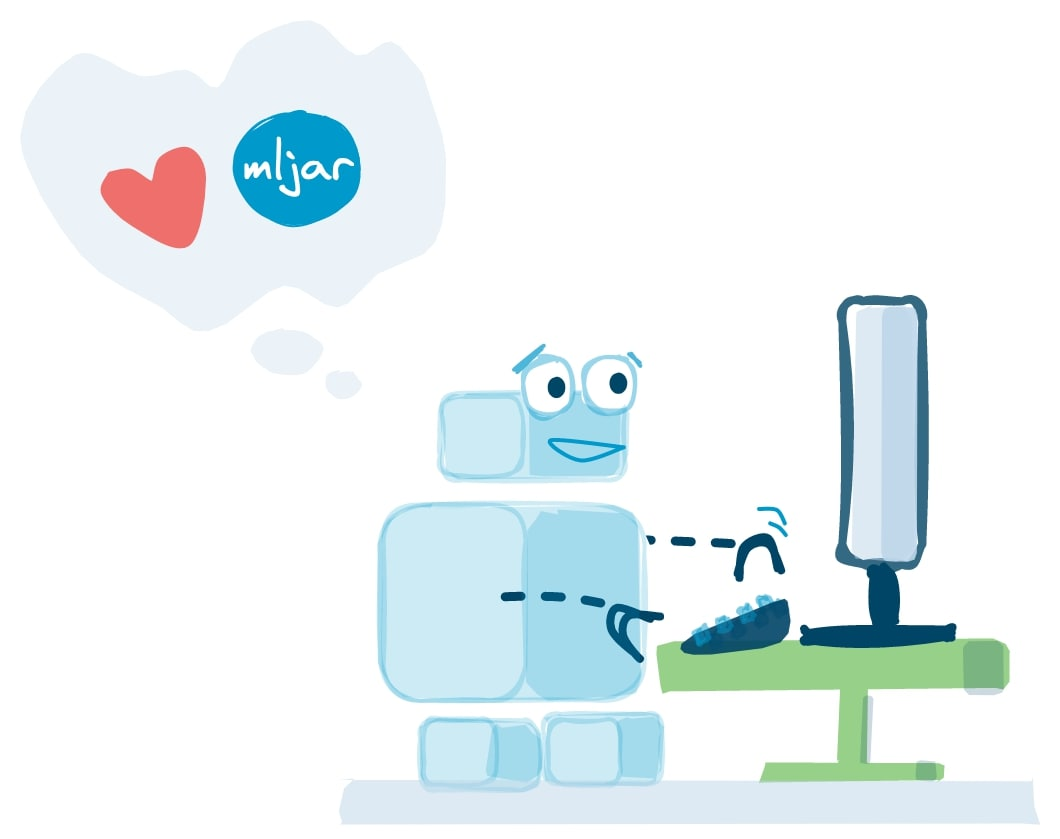In business and science alike, while conducting some processes, it is always necessary to measure efficiency and quality. When it comes to the financial deals, the situation is simple. Income either in short or in long term is the way to go. But what about Machine Learning? To measure the quality of a developed model, we use the process of validation which ensures that we are moving forward in our search for the efficiency and the optimal capacity.

## Data is what you need

To define what validation is, we need to talk about datasets. To develop a machine learning model, we need some data to train on. The data consists of rows of values. Each value corresponds to a given column. Just like in an Excel file or relational SQL database.Above, there is the UCI ML Wine recognition dataset often used in the machine learning tutorials. It was loaded with a usage of Python libraries Scikit Learn and Pandas. As we can see the first 5 rows of the dataset, the samples contain different values which are results of chemical analysis of the wines. They are grouped in different columns or as they are called in Machine Learning: features. Each of them has its own meaning. For example, the alcohol column is the concentration of alcohol in the given sample and the magnesium column is the concentration of magnesium in the given sample.

## Data trinity - train, validation, and test

Now, knowing what we are dealing with, we can move further. First, we need to understand that we need data for the learning. But we also need data for testing our model after the development phase to see if we succeeded. Due to that, we split our dataset into two parts: the train set and the test set. The train set is supposed to be used fully to gain as much predictive power as possible. But the test set is supposed to be left for the final testing. It cannot be used to check the model in the midway of the work as it would leak some information to the model and it would let it perform unnaturally well on the test set. For that reason, we divide the train set further into two parts. One of them is still the train set used for learning and the second is the validation set. It is used to check the performance of the model on the previously unseen examples during the training and the development.

Note: The possible leakage of information is not an algorithmic phenomenon but happens due to our changes to the model’s parameters in the reaction for the results on the test set.## Building intuition

The intuition behind the split is simple. Imagine the time you spent at school on solving mathematical problems. During the classes, you were solving some set of exercises to learn the subject, but after finishing the chapter, the teacher used to give you a test with similar tasks but with some tweaks. These could be different numbers or changed text. And at the end of the school, you took the final exam. It could contain any problems dealing with the subjects you were learning during the part of your education. Now, imagine two things. First, your teacher takes the chapter test tasks from the same set as you were learning before without changing any of them. Some people may just memorize the solutions without understanding them and get a good grade this way. But the grade doesn’t mean that they have learned the subject as they may fail on different tasks from the same chapter. This is why we validate. The second thing to imagine is that the problems from the final exam could be somehow used either during your lessons (training) or chapter test (validation). Thanks to that, you may know how to solve them without really understanding the subject thoroughly. This is why the test set needs to remain separate from the others.

## Measuring the efficiency

Technically speaking, the training is a process of minimizing the loss function. It is sometimes called objective function and quantifies the mistakes our model makes during prediction on the training and the validation data. The lower it is, the better the estimator performs. During the training, we measure the loss function on both the training and the validation sets. The first is taken into account by the algorithm performing the learning process, but the second is left only for consideration of the developer.At first, we can expect that as the model gains performance on the training set it will perform better on the validation as well. But this is not so simple. Here, we approach the concept of capacity. This is the ability of our model to learn more patterns which also can be more complicated. For low capacity, it has problems with learning the different nuances of the data. Some of the details may be totally dropped or squished into the same pattern. That can result with a significantly lower accuracy of the model. This phenomenon is called underfitting. In contrast, high capacity causes the model to learn individual samples instead of patterns encoded among them. This is overfitting. Comparing these two to our previous school analogy, we can say that a student is underfitting if they can’t learn how to solve the problems presented during the classes. By the same analogy, overfitting student is the one who simply memorizes all solutions from the classes and due to that they fail at the test.

This shows why finding the optimal capacity is so important. It enables the best estimation basing on the given data. Obviously, it is not an easy thing to do as it requires a lot of experience and intuition. This is why so much effort is invested into the automatic search of the optimal capacity. It lets the developer rest and automatically conducts the search basing on the returned validation loss value or another metrics. One of the examples is MLJAR. Where the search of parameters is held for the user by an algorithm returning the most optimal solution found.

## How to split

It turns out that taking a proper split of the train test for the learning and the validation parts is an art itself. We have three different approaches to the problem. All of them have some tradeoffs among stability, accuracy and computational time cost.

## Holdout

This is the simplest method. Also called a simple split. We take the training set and divide into two parts by indexes with a given split ratio. For example, with the split ratio rs equal to 0.2 we have 80% of the dataset for the learning process and 20% of the dataset for the validation process. The advantage of this split is low computational cost. Both the training and the validation are run only once and only on the corresponding parts of the set.The problem which often arises with this method is that the dataset can be either sorted according to some classes or certain patterns. In this case, the validation test will have a different statistical distribution than the train test. This can result in a very poor performance. Example of such a problem can be a set containing data about people below the age of 21 with height and age as features and mass as the target for prediction. If we sorted it by age and took children before puberty as the training set, the trained model would have a massive error on the validation test containing the data concerning teenagers after puberty as the relation between mass and height changes a lot with age. A similar problem can occur if we have unbalanced classes in the dataset. Let’s say that we have a dataset of bank transfers and we want to predict which of them is fraudulent. Probably 99% of the transactions are legally correct and after taking the split it can happen that the train set won’t contain any of the fraudulent transactions. Due to that, the model will be able to learn the legal transactions only.

Solution to these problems mentioned above is shuffling the data before taking the split. Thanks to that the distribution across indices will be homogenous and so the train and the validation sets will be statistically representative.

## K-fold

This method is based on the iterative repeating of the holdout method by taking every time a different part of the set for validation purposes. For example, the k=3 case leads to a division of the set into three parts with the same number of samples. Then the model learns on two of the folds and is evaluated on the third. This is repeated three times while taking every time different third fold of the set for validation.This method suffers the same problems as the holdout. Approaching un-homogenous dataset can lead to unexpected differences in performance on the different parts of the set. This is solved either by shuffling the dataset as before or by using the stratified k-fold. The second method instead of dividing the set into three parts chronologically by indexes takes different smaller parts of the set and glues them in a way that results in three parts of the same length. This amounts to some randomness in the splitting what solves the problem at least partially.

Together with some randomness from shuffling or stratifying, this method achieves great stability in measuring the performance. But its downside is the higher computational cost than in the case of the holdout method. For example, taking k=5, we train the model five times on 80% of the set and the same way goes the validation. This gives us five times more computational time comparing to the holdout with the split ratio equal to 0.2.

## Leave one out

Taking the k in the k-fold bigger and bigger leads to the limit of the number of samples in the dataset. This case is the LOO (leave one out) method where we train the model on the whole training set except a chosen sample and validate the model on it. Then, we repeat it for all samples. This gives us a very stable performance of the quality measurement. Obviously, this method is very computationally expensive. The model is trained separately for every sample in the set. Due to that, it is rarely used in practice.

## How MLJAR does it?

For example, in MLJAR while creating a new experiment, we can choose the method of our validation. Below, we can see some results. Validation column tells us what method we have chosen. In this case, it was k-fold for k=3 or k=5 with shuffling. The Metric column shows us the loss function of our choice and the score is the performance of the best model found during the automatic search.## Validation in a nutshell

Concluding, the process of validation is a key element to developing well performing predictive models. It allows us to find the optimal capacity preventing both underfitting and overfitting. But there is still a long way ahead of us in making the process easy. And here come the methods of automatic architecture search.### Convert Python Notebooks to Web Apps

We are working on open-source framework Mercury for converting Jupyter Notebooks to interactive Web Applications.### Articles you might find interesing